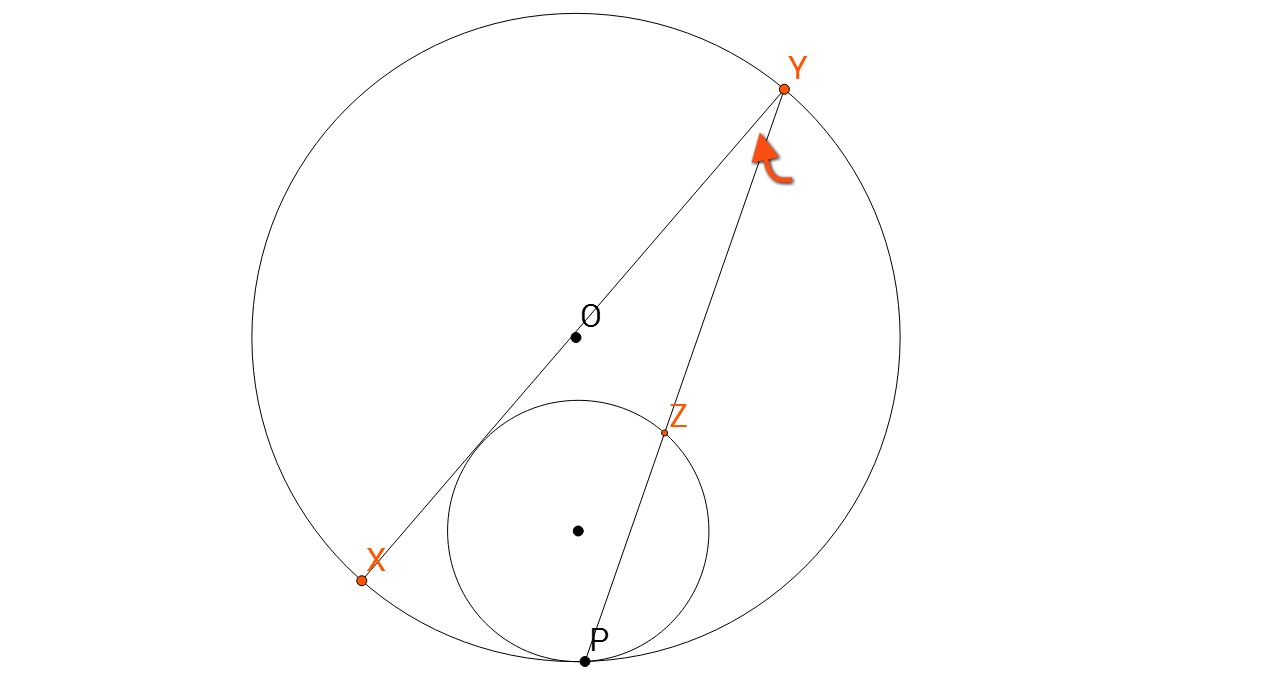# Pre RMO

Geometry Level 4

In the figure below,the smaller circle touches the bigger circle at $P$. The centre of the bigger circle is $O$. Let $XY$ be the diameter of the bigger circle which is also tangent to smaller circle. Let $PY$ intersect the smaller circle at $Z$. If $YZ = 2PZ$, find the magnitue of angle $PYX$ in degrees.

×